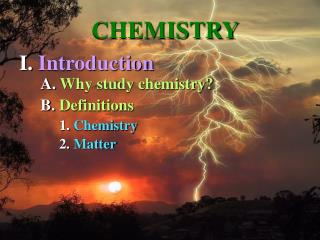# A. Why study chemistry? - PowerPoint PPT PresentationDownload PresentationA. Why study chemistry?

A. Why study chemistry?
Download Presentation## A. Why study chemistry?

- - - - - - - - - - - - - - - - - - - - - - - - - - - E N D - - - - - - - - - - - - - - - - - - - - - - - - - - -
##### Presentation Transcript

1. CHEMISTRY I. Introduction A. Why study chemistry? B. Definitions 1. Chemistry 2. Matter

2. 3. Elements

3. 4. Molecule

4. 5. Compound

5. Figure 2.3

6. Figure 5.3

7. II. Atomic Chemistry A. Particles / Structure

8. Figure 2.5

9. B. Atomic & Mass Number

11. Figure 2.7

12. Isotopes = moreneutrons but stable Radioisotopes = nucleus decay giving off alpha and beta particles, plus gamma rays. Decay = half life Why would this activity be so bad for cells?

13. E. Electrons

14. Figure 2.8 Figure 2.6 Figure 2.9

15. Figure 2.10

16. www.Ausetute.com.au

17. Figure 2.9 Valence => number of electrons in the outermost shell

18. Figure 2.9 Electronegativity degree of attraction for electrons

19. III. Molecular Chemistry A. Definition B. Chemical Bonds 1. Definition 2. Types a. Electron Sharing

20. Non-polar covalent bonds Figure 2.12

21. Polar Figure 2.13

22. ii. Ionic = giving and receiving electrons Figure 2.14 Figure 2.15

23. b. Hydrogen Sharing

24. Hydrogen bonds sharinga hydrogen Figure 2.16

25. Hydrogen bonds sharinga hydrogen Figure 3.2

26. c. VanderWalls Forces

27. VanderWalls Forces sharinga charge

28. C. Formulas & Models 1. Why Important?

29. c. Structural Figure 2.18 Figure 2.17 a. Molecular b. Empirical

30. IV. Chemical Reactions A. Definition B. Types 1. Synthesis, Dehydration, or Anabolic 2. Decomposition, Hydrolytic,or Catabolic 3. Exchange

31. A(OH) + B(H) AB + H2O AB + H2O  A(OH) + B(H) AB + CD  AC + BD

32. C. Factors Affecting Rates

33. V. Inorganic Molecules A. Water 1. Properties

34. States of Water

35. Polar

36. H-Bonding Potential Figure 3.2

37. Density Figure 3.6

38. Cohesive Forces Figure 3.4

39. Cohesive Forces Figure 3.3